# Problem 1 Three samples of fine aggregate (FA) have the following properties Sample Measure Wet Mass (g) Dry Mass (g) Absorption (%) 622.6 591.7 620.9 593 620.0 592.6 2.6 2.52.2 Determine the fol...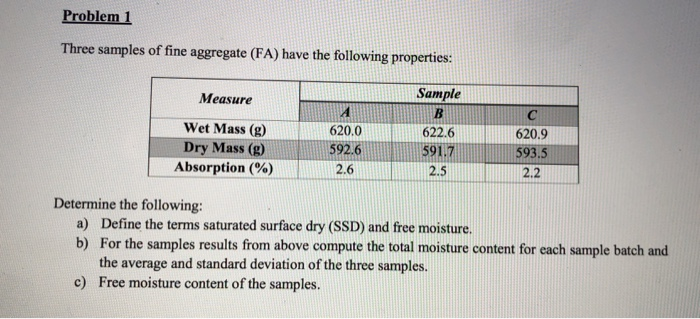Problem 1 Three samples of fine aggregate (FA) have the following properties Sample Measure Wet Mass (g) Dry Mass (g) Absorption (%) 622.6 591.7 620.9 593 620.0 592.6 2.6 2.52.2 Determine the following: a) Define the terms saturated surface dry (SSD) and free moisture. b) For the samples results from above compute the total moisture content for each sample batch and the average and standard deviation of the three samples. Free moisture content of the samples. c)

Saturated surface dry:

It is defined as the condition in aggregates in which the surface is dry but the inner particle void are saturated with water, this condition make no surface adsorption through aggregates.

Free moisture:

It is defined as the moisture not retained or absorbed by the aggregates, also the moisture which can be removed by air drying.

TOTAL MOISTURE CONTENT-

Sample A = 620-592.6 = 27.4g

Sample B = 622.6-591.7 = 30.9g

Sample C= 620.9-593.5 = 27.4g

AVERAGE

FOR WET SAMPLES A, B, & C = 621.1667

FOR DRY SAMPLES A, B, & C = 592.6

STANDARD DEVIATION

FOR WET SAMPLES A, B, & C = 1.320353

FOR DRY SAMPLES A, B, & C = 0.9

FREE MOISTURE CONTENT

SAMPLE A =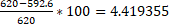SAMPLE B =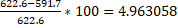SAMPLE C =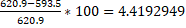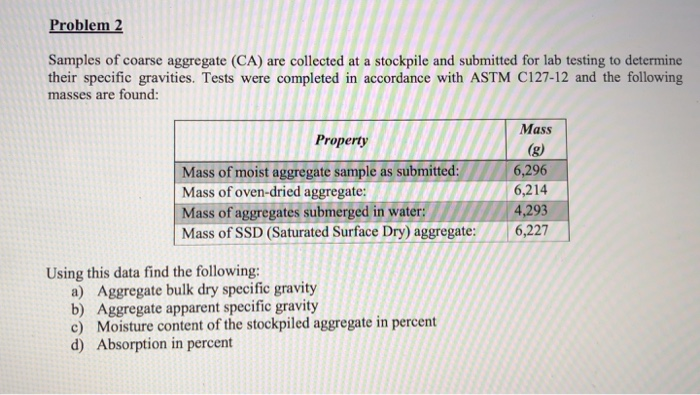Problem 2 Samples of coarse aggregate (CA) are collected at a stockpile and submitted for lab testing to determine their specific gravities. Tests were completed in accordance with ASTM C127-12 and the following masses are found: Mass Property 6,296 6,214 4,293 Mass of moist aggregate sample as submitted: Mass of oven-dried aggregate: Mass of aggregates submerged in water: Mass of SSD (Saturated Surface Dry) aggregate: 6,227 Using this data find the following: a) Aggregate bulk dry specific gravity b) Aggregate...